# Novel, linear, decoupled and unconditionally energy stable numerical methods for the coupled Cahn–Hilliard equations

## Abstract

This paper uses a novel numerical approach to approximate the coupled Cahn–Hilliard equations, which are a highly nonlinear system depicting the phase separation of the homopolymer and copolymer mixtures. The new method is named 3S-IEQ, and its construction and calculation are more straightforward than the invariant energy quadratization and scalar auxiliary variable methods. Notably, we only need to solve two linear decoupled constant-coefficient equations at each time step. Numerical simulations are shown

## Introduction

The phase field model is a mathematical method used to solve interface problem. It is mainly applied to solidification dynamics, but it has also been applied to other situations, such as viscous fingering, crack dynamics, vesicle dynamics, etc. In this method, the boundary conditions at the interface are replaced with partial differential equations to obtain the evolution of the auxiliary field (phase field) as an ordered parameter. The typical phase field models include Allen–Cahn equation, Cahn–Hilliard equation, phase field crystal equation, and Ohta–Kawasaki equation . There are many works devoted to approximating phase field models, such as the nonlinear convex splitting method, the linear stabilized semi-implicit method, the invariant energy quadratization (IEQ) method, and the scalar auxiliary variable (SAV) method .

The coupled Cahn–Hilliard equations are a highly nonlinear system depicting the phase separation of the homopolymer and copolymer mixtures . Avalos  et al. have introduced two phase variables to describe the macro phase separation between homopolymer and copolymer as well as micro phase separation between two components of diblock copolymers, respectively. The authors  have designed two efficient, decoupled, and second-order unconditionally energy stable numerical schemes for this system by utilizing the SAV method.

In this paper, we apply the 3S-IEQ method, which stands for a step-by-step solving approach based on IEQ and constructed by Liu and Chen in , to design three linear, decoupled, and unconditionally energy stable numerical schemes for the coupled Cahn–Hilliard system. The IEQ method sometimes requires specific matrix solvers, such as iteratively solving the elliptic equations with complex variable coefficients at each time step. Although the SAV method does not suffer such a requirement, it is still necessary to solve four fourth-order linear equations at each time step, while we only solve two fourth-order linear equations by 3S-IEQ in terms of the coupled Cahn–Hilliard system.

## Governing equations

We first give some notations, which will be used later. For every $$k\geq 0$$, denote $$(\cdot ,\cdot )_{k}$$ and $$\lVert \cdot \rVert _{k}$$ to be the $$H^{k}(\Omega )$$ inner product and norm, separately. In particular, we abbreviate the $$L^{2}$$ inner product $$(\cdot ,\cdot )_{0}$$ and norm $$\lVert \cdot \rVert _{0}$$ as $$(\cdot ,\cdot )$$ and $$\lVert \cdot \rVert$$, separately. We further define some Sobolev spaces $$L^{2}_{0}(\Omega )=\{u\in L^{2}(\Omega )\vert (u,1)=0\}$$,,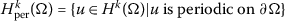.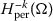is the dual space of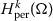. For every $$u\in L^{2}(\Omega )$$, the average value of u in Ω is defined by $$\bar{u}=\frac{1}{|\Omega |}\int _{\Omega}u\,\mathrm{d}\mathbf{x}$$. Assuming $$f\in L^{2}_{0}(\Omega )$$,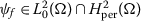is the unique solution of the following elliptic problem:

$$-\Delta \psi _{f} = f\quad \text{in } \Omega .$$
(2.1)

Consequently, we define $$\psi _{f}:=(-\Delta )^{-1}f$$. Assuming $$f,g\in L^{2}_{0}(\Omega )$$, the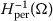inner product and norm can be written as

$$(f,g)_{-1}:=(\nabla \psi _{f},\nabla \psi _{g}),\qquad \Vert f \Vert _{-1}:= \sqrt{(f,f)_{-1}}.$$

Integrating by parts with periodic boundary condition, one has

$$(f,g)_{-1}= \bigl((-\Delta )^{-1}f,g \bigr)= \bigl((- \Delta )^{- \frac{1}{2}}f,(-\Delta )^{-\frac{1}{2}}g \bigr)= \bigl((-\Delta )^{-1}g,f \bigr)=(g,f)_{-1}.$$

Next, we provide a brief description of the coupled Cahn–Hilliard equations proposed in [9, 10], which depict the phase transition of the mixture of a homopolymer and a copolymer. Consider the following Swift–Hohenberg type free energy function:

$$E(u,v)= \int _{\Omega} \biggl(\frac{\varepsilon ^{2}_{u}}{2} \vert \nabla u \vert ^{2}+ \frac{\varepsilon ^{2}_{v}}{2} \vert \nabla v \vert ^{2}+A(u,v)+\frac{\sigma}{2} \bigl\vert (-\Delta )^{-\frac{1}{2}}(v-\bar{v}) \bigr\vert ^{2} \biggr) \,\mathrm{d}\mathbf{x},$$
(2.2)

where Ω is a smooth, open, bounded, connected domain in $$\mathbb{R}^{d}$$ $$(d = 1, 2, 3)$$, $$\varepsilon _{u}$$ and $$\varepsilon _{v}$$ are two real coefficients, proportional to the thickness of the interface between macro phase u and micro phase v. $$A(u,v)=\frac{1}{4}(u^{2}-1)^{2}+\frac{1}{4}(v^{2}-1)^{2}+\alpha uv+ \beta uv^{2}$$, α and β are two coupled parameters. As a consequence, the coupled Cahn–Hilliard equations can be derived by taking the variational derivative of (2.2) in $$H^{-1}(\Omega )$$ with respect to u and v, separately.

$$\textstyle\begin{cases} u_{t} = M_{u}\Delta (-\varepsilon ^{2}_{u} \Delta u+f(u,v) ), \\ v_{t} = M_{v} (\Delta \bigl(-\varepsilon ^{2}_{v}\Delta v+g(u,v) \bigr)-\sigma (v-\bar{v})), \end{cases}$$
(2.3)

where $$M_{u}$$ and $$M_{v}$$ are the motion parameters controlling the moving speed, $$f(u,v)=u^{3}-u+\alpha v+\beta v^{2}$$ and $$g(u,v)=v^{3}-v+\alpha u+2\beta uv$$. Throughout this paper, we assume that all variables satisfy the periodic boundary condition on Ω. Suppose that there is no external forcing other than gravity, and the coupled Cahn–Hilliard equations satisfy the following energy dissipation law and mass conservation law:

\begin{aligned}& \frac{\text{d}}{\text{dt}}E(u,v)=-\frac{1}{M_{u}} \Vert u_{t} \Vert ^{2}_{-1}- \frac{1}{M_{v}} \Vert v_{t} \Vert ^{2}_{-1} \leq 0, \end{aligned}
(2.4)
\begin{aligned}& \frac{\text{d}}{\text{dt}} \int _{\Omega}u\,\text{dt}= \frac{\text{d}}{\text{dt}} \int _{\Omega}v\,\text{dt}=0. \end{aligned}
(2.5)

Before providing the discrete formulations, we let $$N>0$$ be a positive integer. For $$n\leq N$$, set $$\delta t=T/N$$, $$t^{n}=n\delta t$$, $$u^{n}$$ denotes the numerical approximation of $$u(t^{n})$$ and $$u^{n+\frac{1}{2}}=\frac{u^{n+1}+u^{n}}{2}$$.

## Numerical schemes

In this section, we apply the 3S-IEQ method, which was first introduced by Liu and Chen in , to design linear, decoupled, and unconditionally energy stable numerical schemes for the coupled Cahn–Hilliard system. Both the first-order backward Euler (BDF1), the second-order Crank–Nicolson (CN), and the second-order backward differentiation formula (BDF2) methods can be successfully applied to this system. Without losing generality, we mainly focus on the second-order CN scheme. We introduce an auxiliary variable η as follows:

$$\eta := \eta (u,v) = F(u,v)+C = A(u,v)-\frac{S}{2}u^{2}- \frac{S}{2}v^{2}+C,$$
(3.1)

where $$S\geq 0$$ is a stable constant, and C is a real constant to impose $$F(u,v)+C\neq 0$$. It is obvious to see that $$F(u,v)+C\neq 0$$ is more effective than $$F(u,v)+C\geq 0$$. Then, we define the following new functions $$\mathcal{H}(u,v,\eta )$$ and $$\mathcal{G}(u,v,\eta )$$ which are equivalent to the nonlinear functional $$f(u,v)$$ and $$g(u,v)$$.

$$\mathcal{H}(u,v,\eta ) = \frac{\eta}{F(u,v)+C}f(u,v),\qquad \mathcal{G}(u,v,\eta ) = \frac{\eta}{F(u,v)+C}g(u,v).$$
(3.2)

Thus, the original coupled Cahn–Hilliard system (2.3) can be rewritten as

\begin{aligned} &u_{t} = M_{u}\Delta \bigl(- \varepsilon ^{2}_{u}\Delta u+Su+ \mathcal{H}(u,v,\eta ) \bigr), \end{aligned}
(3.3a)
\begin{aligned} &v_{t} = M_{v} \bigl(\Delta \bigl(- \varepsilon ^{2}_{v}\Delta v+Sv+ \mathcal{G}(u,v,\eta ) \bigr)-\sigma (v-\bar{v}) \bigr), \end{aligned}
(3.3b)
\begin{aligned} &\eta _{t} = \mathcal{H}(u,v,\eta )u_{t}+\mathcal{G}(u,v,\eta )v_{t}. \end{aligned}
(3.3c)

By taking the $$L^{2}$$ inner product of (3.3a) and (3.3b) with $$(-\Delta )^{-1}u_{t}$$ and $$(-\Delta )^{-1}v_{t}$$, we can obtain the following modified energy dissipation law:

$$\frac{\text{d}}{\text{dt}}\mathcal{E}(u,v,\eta ) = - \frac{1}{M_{u}} \Vert u_{t} \Vert ^{2}_{-1}- \frac{1}{M_{v}} \Vert v_{t} \Vert ^{2}_{-1} \leq 0,$$
(3.4)

where

\begin{aligned} \mathcal{E}(u,v,\eta ) =& \int _{\Omega} \biggl( \frac{\varepsilon _{u}}{2} \vert \nabla u \vert ^{2}+\frac{\varepsilon _{v}}{2} \vert \nabla v \vert ^{2}+\frac{S}{2} \vert u \vert ^{2}+ \frac{S}{2} \vert v \vert ^{2} \\ &{}+\frac{\sigma}{2} \bigl\vert (-\Delta )^{-\frac{1}{2}}(v-\bar{v}) \bigr\vert ^{2}+ \eta -C \biggr)\,\mathrm{d}\mathbf{x}. \end{aligned}
(3.5)

### Scheme 1

(CN)

Assuming that $$(u^{n-1}, v^{n-1}, \eta ^{n-1})$$ and $$(u^{n}, v^{n}, \eta ^{n})$$ are already calculated with $$n\geq 1$$, we then update $$(u^{n+1}, v^{n+1}, \eta ^{n+1})$$ as follows:

\begin{aligned} &\frac{u^{n+1}-u^{n}}{M_{u}\delta t} = \Delta \bigl(-\varepsilon ^{2}_{u} \Delta u^{n+\frac{1}{2}}+Su^{n+\frac{1}{2}}+ \mathcal{H}^{*,n+ \frac{1}{2}} \bigr), \end{aligned}
(3.6a)
\begin{aligned} &\frac{v^{n+1}-v^{n}}{M_{v}\delta t} = \Delta \bigl(-\varepsilon ^{2}_{v} \Delta v^{n+\frac{1}{2}}+Sv^{n+\frac{1}{2}}+ \mathcal{G}^{*,n+ \frac{1}{2}} \bigr)-\sigma \bigl(v^{n+\frac{1}{2}}- \bar{v}^{n+\frac{1}{2}}\bigr), \end{aligned}
(3.6b)
\begin{aligned} &\eta ^{n+1}-\eta ^{n} = \mathcal{H}^{*,n+\frac{1}{2}}\bigl(u^{n+1}-u^{n}\bigr)+ \mathcal{G}^{*,n+\frac{1}{2}}\bigl(v^{n+1}-v^{n}\bigr), \end{aligned}
(3.6c)

where

\begin{aligned}& \mathcal{H}^{*,n+\frac{1}{2}}=\mathcal{H}\bigl(u^{*,n+\frac{1}{2}},v^{*,n+ \frac{1}{2}}, \eta ^{*,n+\frac{1}{2}}\bigr),\qquad \mathcal{G}^{*,n+ \frac{1}{2}}=\mathcal{G} \bigl(u^{*,n+\frac{1}{2}},v^{*,n+\frac{1}{2}}, \eta ^{*,n+\frac{1}{2}}\bigr), \\& u^{*,n+\frac{1}{2}}=\frac{3u^{n}-u^{n-1}}{2}, \qquad v^{*,n+ \frac{1}{2}}= \frac{3v^{n}-v^{n-1}}{2}, \qquad \eta ^{*,n+\frac{1}{2}}= \frac{3\eta ^{n}-\eta ^{n-1}}{2}. \end{aligned}

### Theorem 3.1

Scheme (3.6a)(3.6c) is unconditionally energy stable satisfying the following discrete energy dissipation law:

$$\mathcal{E}_{cn}^{n+1}- \mathcal{E}_{cn}^{n} =-\frac{1}{M_{u}\delta t} \bigl\Vert u^{n+1}-u^{n} \bigr\Vert ^{2}_{-1}- \frac{1}{M_{v}\delta t} \bigl\Vert v^{n+1}-v^{n} \bigr\Vert ^{2}_{-1} \leq 0,$$
(3.7)

where

\begin{aligned} \mathcal{E}_{cn}^{n} =& \frac{1}{2} \bigl(\varepsilon ^{2}_{u} \bigl\Vert \nabla u^{n} \bigr\Vert ^{2}+\varepsilon ^{2}_{v} \bigl\Vert \nabla v^{n} \bigr\Vert ^{2}+S \bigl\Vert u^{n} \bigr\Vert ^{2}+S \bigl\Vert v^{n} \bigr\Vert ^{2}+ \sigma \bigl\Vert v^{n}-\bar{v}^{n} \bigr\Vert ^{2}_{-1} \bigr) \\ &{}+ \int _{\Omega} \eta ^{n}\,\mathrm{d}\mathbf{x}-C \vert \Omega \vert . \end{aligned}
(3.8)

### Proof

Firstly, applying $$\Delta ^{-1}$$ to (3.6a) and (3.6b), we have

$$\Delta ^{-1}\frac{u^{n+1}-u^{n}}{M_{u}\delta t} = -\varepsilon ^{2}_{u} \Delta u^{n+\frac{1}{2}}+Su^{n+\frac{1}{2}}+ \mathcal{H}^{*,n+ \frac{1}{2}}$$
(3.9)

and

$$\Delta ^{-1}\frac{v^{n+1}-v^{n}}{M_{v}\delta t} = -\varepsilon ^{2}_{v} \Delta v^{n+\frac{1}{2}}+Sv^{n+\frac{1}{2}}+ \mathcal{G}^{*,n+ \frac{1}{2}}-\sigma \Delta ^{-1}\bigl(v^{n+\frac{1}{2}}- \bar{v}^{n+ \frac{1}{2}}\bigr).$$
(3.10)

Secondly, taking the $$L^{2}$$ inner product of the above two equations with $$u^{n+1}-u^{n}$$ and $$v^{n+1}-v^{n}$$, one has

\begin{aligned} -\frac{1}{M_{u}\delta t} \bigl\Vert u^{n+1}-u^{n} \bigr\Vert ^{2}_{-1} =& \frac{1}{2} \varepsilon ^{2}_{u} \bigl( \bigl\Vert \nabla u^{n+1} \bigr\Vert ^{2}- \bigl\Vert \nabla u^{n} \bigr\Vert ^{2} \bigr)+ \frac{S}{2} \bigl( \bigl\Vert u^{n+1} \bigr\Vert ^{2}- \bigl\Vert u^{n} \bigr\Vert ^{2} \bigr) \\ &{}+ \bigl(\mathcal{H}^{*,n+\frac{1}{2}},u^{n+1}-u^{n} \bigr) \end{aligned}
(3.11)

and

\begin{aligned} -\frac{1}{M_{v}\delta t} \bigl\Vert v^{n+1}-v^{n} \bigr\Vert ^{2}_{-1} = &\frac{1}{2}\varepsilon ^{2}_{v} \bigl( \bigl\Vert \nabla v^{n+1} \bigr\Vert ^{2}- \bigl\Vert \nabla v^{n} \bigr\Vert ^{2} \bigr) \\ &{}+ \frac{S}{2} \bigl( \bigl\Vert v^{n+1} \bigr\Vert ^{2}- \bigl\Vert v^{n} \bigr\Vert ^{2} \bigr)+ \bigl(\mathcal{G}^{*,n+\frac{1}{2}},v^{n+1}-v^{n} \bigr) \\ &{}+\sigma \bigl((-\Delta )^{-1} \bigl(v^{n+\frac{1}{2}}- \bar{v}^{n+ \frac{1}{2}} \bigr),v^{n+1}-v^{n} \bigr). \end{aligned}
(3.12)

Thirdly, integrating (3.6c) once, we derive that

$$\int _{\Omega}\eta ^{n+1}\mathrm{d}\mathbf{x}- \int _{\Omega}\eta ^{n} \mathrm{d}\mathbf{x}= \bigl( \mathcal{H}^{*,n+\frac{1}{2}},u^{n+1}-u^{n} \bigr) + \bigl( \mathcal{G}^{*,n+\frac{1}{2}},v^{n+1}-v^{n} \bigr).$$
(3.13)

Moreover, by integrating (3.10) once, we obtain the mass conservation law for v which reads $$\int _{\Omega}v^{n+1}\mathrm{d}\mathbf{x}=\int _{\Omega}v^{n}\mathrm{d}\mathbf{x}$$. Thus, we can split $$v^{n+1}-v^{n}$$ as $$(v^{n+1}-\bar{v}^{n+1})-(v^{n}-\bar{v}^{n})$$. On the other hand, $$\int _{\Omega}(v^{n+\frac{1}{2}}-\bar{v}^{n+\frac{1}{2}}) \mathrm{d}\mathbf{x}=0$$, $$v^{n+\frac{1}{2}}-\bar{v}^{n+\frac{1}{2}}\in L^{2}_{0}(\Omega )$$, so we derive that

$$\sigma \bigl((-\Delta )^{-1} \bigl(v^{n+\frac{1}{2}}- \bar{v}^{n+ \frac{1}{2}} \bigr),v^{n+1}-v^{n} \bigr) = \frac{\sigma}{2} \bigl( \bigl\Vert v^{n+1}-\bar{v}^{n+1} \bigr\Vert ^{2}_{-1}- \bigl\Vert v^{n}- \bar{v}^{n} \bigr\Vert ^{2}_{-1} \bigr).$$
(3.14)

Finally, combining (3.11), (3.12), (3.13), and (3.14), we obtain

\begin{aligned}[b] &-\frac{1}{M_{u}\delta t} \bigl\Vert u^{n+1}-u^{n} \bigr\Vert ^{2}_{-1}- \frac{1}{M_{v}\delta t} \bigl\Vert v^{n+1}-v^{n} \bigr\Vert ^{2}_{-1} \\ &\quad = \frac{1}{2}\varepsilon ^{2}_{u} \bigl( \bigl\Vert \nabla u^{n+1} \bigr\Vert ^{2}- \bigl\Vert \nabla u^{n} \bigr\Vert ^{2} \bigr) + \frac{S}{2} \bigl( \bigl\Vert u^{n+1} \bigr\Vert ^{2}- \bigl\Vert u^{n} \bigr\Vert ^{2} \bigr)+ \int _{\Omega}\eta ^{n+1}\mathrm{d}\mathbf{x}- \int _{\Omega} \eta ^{n}\mathrm{d}\mathbf{x} \\ &\qquad {}+\frac{1}{2}\varepsilon ^{2}_{v} \bigl( \bigl\Vert \nabla v^{n+1} \bigr\Vert ^{2}- \bigl\Vert \nabla v^{n} \bigr\Vert ^{2} \bigr)+ \frac{S}{2} \bigl( \bigl\Vert v^{n+1} \bigr\Vert ^{2}- \bigl\Vert v^{n} \bigr\Vert ^{2} \bigr)\\ &\qquad {}+\frac{\sigma}{2} \bigl( \bigl\Vert v^{n+1}- \bar{v}^{n+1} \bigr\Vert ^{2}_{-1}- \bigl\Vert v^{n}-\bar{v}^{n} \bigr\Vert ^{2}_{-1} \bigr), \end{aligned}

which directly complete this proof. □

### Scheme 2

(BDF2)

Assuming that $$(u^{n-1}, v^{n-1}, \eta ^{n-1})$$ and $$(u^{n}, v^{n}, \eta ^{n})$$ are already calculated with $$n\geq 1$$, we then update $$(u^{n+1}, v^{n+1}, \eta ^{n+1})$$ as follows:

\begin{aligned} &\frac{3u^{n+1}-4u^{n}+u^{n-1}}{2M_{u}\delta t} = \Delta \bigl(- \varepsilon ^{2}_{u}\Delta u^{n+1}+Su^{n+1}+ \mathcal{H}^{**,n+1} \bigr), \end{aligned}
(3.15a)
\begin{aligned} &\frac{3v^{n+1}-4v^{n}+v^{n-1}}{2M_{v}\delta t} = \Delta \bigl(- \varepsilon ^{2}_{v}\Delta v^{n+1}+Sv^{n+1}+ \mathcal{G}^{**,n+1} \bigr)-\sigma \bigl(v^{n+1}- \bar{v}^{n+1}\bigr), \end{aligned}
(3.15b)
\begin{aligned} &3\eta ^{n+1}-4\eta ^{n}+\eta ^{n-1} \\ &\quad = \mathcal{H}^{**,n+1}\bigl(3u^{n+1}-4u^{n}+u^{n-1} \bigr)+ \mathcal{G}^{**,n+1}\bigl(3v^{n+1}-4v^{n}+v^{n-1} \bigr), \end{aligned}
(3.15c)

where

\begin{aligned}& \mathcal{H}^{**,n+1}=\mathcal{H}\bigl(u^{**,n+1},v^{**,n+1}, \eta ^{**,n+1}\bigr), \qquad \mathcal{G}^{**,n+1}=\mathcal{G} \bigl(u^{**,n+1},v^{**,n+1},\eta ^{**,n+1}\bigr), \\& u^{**,n+1}=2u^{n}-u^{n-1}, \qquad v^{**,n+1}=2v^{n}-v^{n-1}, \qquad \eta ^{**,n+1}=2\eta ^{n}-\eta ^{n-1}. \end{aligned}

### Theorem 3.2

Scheme (3.15a)(3.15c) is unconditionally energy stable satisfying the following discrete energy dissipation law:

\begin{aligned} \mathcal{E}_{bdf2}^{n+1,n}- \mathcal{E}_{bdf2}^{n,n-1} =&- \frac{1}{2M_{u}\delta t} \bigl\Vert 3u^{n+1}-4u^{n}+u^{n-1} \bigr\Vert ^{2}_{-1}- \frac{1}{2M_{v}\delta t} \bigl\Vert 3v^{n+1}-4v^{n}+v^{n-1} \bigr\Vert ^{2}_{-1} \\ \leq &0, \end{aligned}
(3.16)

where

\begin{aligned}[b] \mathcal{E}_{bdf2}^{n,n-1} ={}& \frac{\varepsilon ^{2}_{u}}{4} \bigl( \bigl\Vert \nabla u^{n} \bigr\Vert ^{2}+ \bigl\Vert \nabla \bigl(2u^{n}-u^{n-1} \bigr) \bigr\Vert ^{2} \bigr)+\frac{S}{4} \bigl( \bigl\Vert u^{n} \bigr\Vert ^{2}+ \bigl\Vert 2u^{n}-u^{n-1} \bigr\Vert ^{2} \bigr) \\ &{}+\frac{\varepsilon ^{2}_{v}}{4} \bigl( \bigl\Vert \nabla v^{n} \bigr\Vert ^{2}+ \bigl\Vert \nabla \bigl(2v^{n}-v^{n-1} \bigr) \bigr\Vert ^{2} \bigr)+\frac{S}{4} \bigl( \bigl\Vert v^{n} \bigr\Vert ^{2}+ \bigl\Vert 2v^{n}-v^{n-1} \bigr\Vert ^{2} \bigr) \\ &{}+\frac{\sigma}{4} \bigl( \bigl\Vert v^{n}- \bar{v}^{n} \bigr\Vert ^{2}_{-1}+ \bigl\Vert 2\bigl(v^{n}- \bar{v}^{n}\bigr)- \bigl(v^{n-1}-\bar{v}^{n-1}\bigr) \bigr\Vert ^{2}_{-1} \bigr) \\ &{}+\frac{\sigma}{2} \int _{\Omega}\bigl(3\eta ^{n}-\eta ^{n-1}\bigr) \,\mathrm{d}\mathbf{x}-C \vert \Omega \vert . \end{aligned}
(3.17)

### Proof

The proof of this theorem is similar to Theorem 3.1. Indeed, we rely on the identity $$2a(3a-4b+c)=a^{2}-b^{2}+(2a-b)^{2}-(2b-c)^{2}+(a-2b+c)^{2}$$. So we omit the proof. □

### Theorem 3.3

The second-order Scheme 1and Scheme 2are uniquely solvable. $$(u^{n+1}, v^{n+1}, \eta ^{n+1})$$ can be solved step by step, the fast Fourier transform is highly efficient for solving the fully discrete schemes.

### Proof

Taking the second-order CN Scheme 1 as an example, the proof of BDF2 Scheme 2 is similar. We rewrite Scheme 1 as the following formulations:

\begin{aligned} & \biggl(1+\frac{1}{2}\delta tM_{u}\varepsilon ^{2}_{u} \Delta ^{2}-\frac{1}{2}\delta tM_{u}S\Delta \biggr)u^{n+1} \\ &\quad = u^{n}- \frac{1}{2}\delta tM_{u}\varepsilon ^{2}_{u}\Delta ^{2}u^{n}+ \frac{1}{2}\delta tM_{u}S\Delta u^{n}+\delta tM_{u}\Delta \mathcal{H}^{*,n+ \frac{1}{2}}, \\ & \biggl(1+\frac{1}{2}\delta tM_{v}\varepsilon ^{2}_{v}\Delta ^{2}- \frac{1}{2} \delta tM_{v}S\Delta +\frac{1}{2}\delta tM_{v} \sigma \biggr)v^{n+1} \\ &\quad = v^{n}-\frac{1}{2}\delta tM_{v}\varepsilon ^{2}_{v} \Delta ^{2}v^{n}+\frac{1}{2}\delta tM_{v}S\Delta v^{n} \\ &\qquad {}+ \delta tM_{v}\Delta \mathcal{G}^{*,n+\frac{1}{2}} -\frac{1}{2} \delta tM_{v} \sigma v^{n} + \delta tM_{v} \sigma \bar{v}^{n}. \end{aligned}
(3.18)

The two linear systems are uniquely solvable because the coefficient matrices are not symmetric positive definite but constant coefficient matrices. Meanwhile, $$u^{n+1}$$, $$v^{n+1}$$, and $$\eta ^{n+1}$$ can be solved totally decoupled. We can first calculate $$u^{n+1}$$, $$v^{n+1}$$, then $$\eta ^{n+1}$$ can be updated by

$$\eta ^{n+1} = \eta ^{n}+\mathcal{H}^{*,n+\frac{1}{2}} \bigl(u^{n+1}-u^{n}\bigr)+ \mathcal{G}^{*,n+\frac{1}{2}} \bigl(v^{n+1}-v^{n}\bigr).$$

□

### Remark 3.1

In , the authors proposed two time second-order schemes based on the CN and the BDF2 methods. Although the second-order schemes derived by SAV are decoupled and unconditionally energy stable, the authors need to solve four fourth-order linear equations with constant coefficients at each time step. Here we only solve two fourth-order linear equations that significantly improve the efficiency.

### Remark 3.2

Both the second-order Scheme 1 and Scheme 2 include three time levels, and $$(u^{n+1},v^{n+1},\eta ^{n+1})$$ can be updated recursively by the recurrence relation as long as the initial values $$(u^{0},v^{0},\eta ^{0})$$ and $$(u^{1},v^{1},\eta ^{1})$$ have been calculated. The former is directly derived by initial conditions, and the latter is computed by BDF1.

### Scheme 3

(BDF1)

Assuming that $$(u^{n}, v^{n}, \eta ^{n})$$ are already calculated with $$n\geq 0$$, we then update $$(u^{n+1}, v^{n+1}, \eta ^{n+1})$$ as follows:

\begin{aligned} &\frac{u^{n+1}-u^{n}}{M_{u}\delta t} = \Delta \bigl(-\varepsilon ^{2}_{u} \Delta u^{n+1}+Su^{n+1}+ \mathcal{H}\bigl(u^{n},v^{n},\eta ^{n} \bigr) \bigr), \end{aligned}
(3.19a)
\begin{aligned} &\frac{v^{n+1}-v^{n}}{M_{v}\delta t} = \Delta \bigl(-\varepsilon ^{2}_{v} \Delta v^{n+1}+Sv^{n+1}+ \mathcal{G}\bigl(u^{n},v^{n},\eta ^{n} \bigr) \bigr)- \sigma \bigl(v^{n+1}-\bar{v}^{n+1}\bigr), \end{aligned}
(3.19b)
\begin{aligned} &\eta ^{n+1}-\eta ^{n} = \mathcal{H} \bigl(u^{n},v^{n},\eta ^{n}\bigr) \bigl(u^{n+1}-u^{n}\bigr)+ \mathcal{G} \bigl(u^{n},v^{n},\eta ^{n}\bigr) \bigl(v^{n+1}-v^{n}\bigr). \end{aligned}
(3.19c)

Here, the corresponding discrete energy is denoted by

\begin{aligned} \mathcal{E}_{bdf1}^{n} =& \frac{1}{2} \bigl(\varepsilon ^{2}_{u} \bigl\Vert \nabla u^{n} \bigr\Vert ^{2}+\varepsilon ^{2}_{v} \bigl\Vert \nabla v^{n} \bigr\Vert ^{2}+S \bigl\Vert u^{n} \bigr\Vert ^{2}+S \bigl\Vert v^{n} \bigr\Vert ^{2}+ \sigma \bigl\Vert v^{n}-\bar{v}^{n} \bigr\Vert ^{2}_{-1} \bigr) \\ &{}+ \int _{\Omega}\eta ^{n}\mathrm{d}\mathbf{x}-C \vert \Omega \vert . \end{aligned}
(3.20)

## Numerical experiments

We now present various two-dimensional numerical simulations for the coupled Cahn–Hilliard equations. Usually, the proposed time discretization schemes can be combined with any spatial discretization, provided the spatial discretization reaches the desired tolerance error. In this paper, we assume that all variables satisfy the periodic boundary condition and merely focus on time discretization. Therefore, we can apply the Fourier spectral method for spatial discretization, and the fast Fourier transform is easy to solve the fully discrete schemes.

### Temporal convergence test

We test the convergence rates of the three proposed schemes. The parameters are $$\varepsilon _{u}^{2}=\varepsilon _{v}^{2}=0.1$$, $$M_{u}=M_{v}=1$$, $$\alpha =2$$, $$\beta =3$$, $$\sigma =50$$, $$S=0$$. We choose suitable exact solution

$$u(x,y,t)=v(x,y,t)=0.25\sin (x)\sin (y)\cos (t)+0.1.$$
(4.1)

The computational domain is $$\Omega =[-3*\pi , \pi ]^{2}$$, and we use $$128\times 128$$ Fourier modes. In Table 1 and Table 2, we list the $$L^{2}$$ errors for u and v at final time $$T=0.05$$ with different time step sizes $$\delta t=0.005/2^{k}$$, $$k=0,1,\ldots,5$$. These exact solutions and parameters are completely consistent with those in  for convenient comparison.

From Table 1 and Table 2, we can observe that all schemes reach the desired order of accuracy in time. BDF1 is first-order accurate in time, while CN and BDF2 are second-order accurate in time. Compared with the results of , the error is a little bit bigger because 3S-IEQ is a total explicit method.

### Energy stability test and phase transition

We select the following smooth initial conditions to demonstrate the energy stability of the proposed schemes:

$$u(x,y,0) = \sin \bigl(2x(x-1)y(y-1)\bigr),\qquad v(x,y,0) = \cos \bigl(10x(x-1)\bigr)y(y-1),$$
(4.2)

where $$\Omega =[0,1]^{2}$$, and we use $$128\times 128$$ Fourier modes for spatial discretization. From Fig. 1, the original energy E, the modified energy $$\mathcal{E}$$, the discrete energies $$\mathcal{E}_{bdf1}$$, $$\mathcal{E}_{cn}$$, and $$\mathcal{E}_{bdf2}$$ are nonincreasing in time. In Fig. 2, we plot the evolution curves of modified discrete energies $$\mathcal{E}_{bdf1}$$, $$\mathcal{E}_{cn}$$, and $$\mathcal{E}_{bdf2}$$, which are calculated by BDF1, CN, and BDF2 for various time steps. Overall, the schemes developed in this paper are unconditionally energy stable. For the same time step, the discrete energy of CN scheme can obtain a better approximation, especially $$\delta t=0.001, 0.0005, 0.0001$$, which is consistent with the results in . Moreover, Fig. 2 provides a basis for us to select an appropriate time step when simulating the long-term phase transition process, that is, $$\delta t\leq 0.001$$. Figure 3 displays the snapshots of the phase variables u and v at different times. Moreover, we can see that u describes a macro phase separation between homopolymer and copolymer, while v describes a micro phase separation that occurs within the separate domain. Significantly, we choose the same parameters and initial data as  to demonstrate the consistent snapshots of u and v.

In Table 3, we give the CPU time consumed by 3S-IEQ compared with the SAV method in . The three schemes of the former method take significantly less time than the latter, especially for the minimal time step simulation $$\delta t = 0.0001$$, because the authors in  needed to solve four fourth-order linear equations with constant coefficient at each time step. Here we only solve two fourth-order linear equations, which greatly improves the efficiency.

## Conclusions

This paper applies the 3S-IEQ method to design three linear, decoupled, and unconditionally energy stable numerical schemes for the coupled Cahn–Hilliard system. Numerical simulations are shown to demonstrate the time accuracy and unconditional energy stability. The most significant advantage of this method is that we only solve two fourth-order linear equations, which dramatically improves the efficiency, and it can be applied to various gradient flows, including $$L^{2}$$ and $$H^{-1}$$ gradient flows. However, the error and convergence analysis of the proposed schemes is still an open challenge due to the coupling and nonlocality. We will carefully consider the bottleneck in the future.

## Availability of data and materials

Data sharing not applicable to this article as no datasets were generated or analysed during the current study.

## References

1. Allen, S.M., Cahn, J.W.: A microscopic theory for antiphase boundary motion and its application to antiphase domain coarsening. Acta Metall. 27, 1085–1095 (1979)

2. Cahn, J.W., Hilliard, J.E.: Free energy of a non-uniform system. 1. Interfacial free energy. J. Chem. Phys. 28, 258–267 (1958)

3. Elder, K.R., Katakowski, M., Haataja, M., Grant, M.: Modeling elasticity in crystal growth. Phys. Rev. Lett. 88, 245701 (2002)

4. Ohta, T., Kawasaki, K.: Equilibrium morphology of block copolymer melts. Macromolecules 19, 2621–2632 (1986)

5. Gu, S., Zhou, X.: Convex splitting method for the calculation of transition states of energy functional. J. Comput. Phys. 353, 417–434 (2018)

6. Zhang, H., Jiang, X., Zeng, F., Karniadakis, G.E.: A stabilized semi-implicit Fourier spectral method for nonlinear space-fractional reaction-diffusion equations. J. Comput. Phys. 405, 109141 (2019)

7. Li, Q., Mei, L.Q., Yang, X.F., Li, Y.B.: Efficient numerical schemes with unconditional energy stabilities for the modified phase field crystal equation. Adv. Comput. Math. 45, 1551–1580 (2019)

8. Li, Q., Li, X., Yang, X.F., Mei, L.Q.: Highly efficient and linear numerical schemes with unconditional energy stability for the anisotropic phase-field crystal model. J. Comput. Appl. Math. 383, 113122 (2021)

9. Avalos, E., Higuchi, T., Teramoto, T., Yabu, H., Nishiura, Y.: Correction: Frustrated phases under three-dimensional confinement simulated by a set of coupled Cahn–Hilliard equations. Soft Matter 12, 5905–5914 (2016)

10. Avalos, E., Teramoto, T., Komiyama, H., Yabu, H., Nishiura, Y.: Transformation of block copolymer nanoparticles from ellipsoids with striped lamellae into onionlike spheres and dynamical control via coupled Cahn-Hilliard equations. ACS Omega 3, 1304–1314 (2018)

11. Li, Q., Mei, L.: Efficient, decoupled and second-order unconditionally energy stable numerical scheme for the coupled Cahn-Hilliard system in copolymer/hemopolymer mixtures. Comput. Phys. Commun. 260, 107290 (2021)

12. Liu, Z.G., Chen, S.S.: Novel linear decoupled and unconditionally energy stable numerical methods for the modified phase field crystal model. Appl. Numer. Math. 163, 1–14 (2021)

## Acknowledgements

We would like to thank Prof. Mancang Wang at Northwest University for his constructive suggestion.

## Funding

Yongsheng Yan is supported by the National Social Science Foundation of China (21AJY011).

## Author information

Authors

### Contributions

The authors, viz LL, YY, FY, and XM with the consultation of each other carried out this work and drafted the manuscript together. All authors read and approved the final manuscript.

### Corresponding author

Correspondence to Xiaoqiang Ma.

## Ethics declarations

### Competing interests

The authors declare that they have no competing interests.

## Rights and permissions